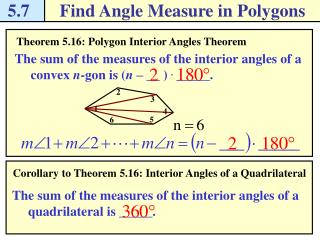DownloadDownload Presentation5.7

# 5.7

Download Presentation## 5.7

- - - - - - - - - - - - - - - - - - - - - - - - - - - E N D - - - - - - - - - - - - - - - - - - - - - - - - - - -
##### Presentation Transcript

1. 5.7 Find Angle Measure in Polygons 2 3 1 4 5 6 Theorem 5.16: Polygon Interior Angles Theorem The sum of the measures of the interior angles of a convex n-gon is (n – __ ) . _____. Corollary to Theorem 5.16: Interior Angles of a Quadrilateral The sum of the measures of the interior angles of a quadrilateral is _____.

2. 5.7 Find Angle Measure in Polygons Theorem 5.16: Polygon Exterior Angles Theorem The sum of the measures of the exterior angles of a convex polygon, one angle at each vertex, is _____. 3 2 4 1 5

3. 5.7 Find Angle Measure in Polygons Find the sum of angle measures in a polygon Example 1 Find the sum of the measure of the interior angles of a convex octagon. Solution An octagon has ____ sides. Use the Polygon Interior Angles Theorem. Substitute __ for n. Subtract. Multiply. The sum of the measures of the interior angles of an octagon is _______.

4. 5.7 Find Angle Measure in Polygons Checkpoint. Complete the following exercises. • Find the sum of the measure of the interior angles of the convex decagon.

5. 5.7 Find Angle Measure in Polygons Find the number of sides of a polygon Example 2 The sum of the measures of the interior angles of a convex polygon is 1260o. Classify the polygon by the number of sides. Solution Use the Polygon Interior Angles Theorem to write an equation involving the number of sides n. Then solve the equation to find the number of sides. Polygon Interior Angle Theorem. Divide each side by ___. Add __ to each side. The polygon has ___ sides. It is a _________.

6. 5.7 Find Angle Measure in Polygons Find an unknown interior angle measure Example 3 Find the value of x in the diagram shown. Solution The polygon is a quadrilateral. Use the Corollary to the Polygon Interior Angles Theorem to write an equation involving x. Then solve the equation. Corollary to Theorem 5.16. Combine like terms. Subtract ____ from each side.

7. 5.7 Find Angle Measure in Polygons Checkpoint. Complete the following exercises. • The sum of the measures of the interior angles of a convex polygon is 1620o. Classify the polygon by the number of sides.

8. 5.7 Find Angle Measure in Polygons N J M K L Checkpoint. Complete the following exercises. • Use the diagram at the right.

9. 5.7 Find Angle Measure in Polygons Find unknown exterior angle measures Example 4 Find the value of x in the diagram shown. Solution Use the Polygon Exterior Angles Theorem to write and solve an equation. Polygon Exterior Angle Theorem. Combine like terms. Solve for x.

10. 5.7 Find Angle Measure in Polygons Checkpoint. Complete the following exercises. • A convex pentagon has exterior angles with measures 66o, 77o, 82o, and 62o. What is the measure of an exterior angle at the fifth vertex.

11. 5.7 Find Angle Measure in Polygons Checkpoint. Complete the following exercises. • Find the measure of (a) each interior angle and (b) each exterior angle of a regular nonagon.

12. 5.7 Find Angle Measure in Polygons Pg. 312, 5.7 #1-40